## Authors

• André Araújo andrealexaraujo@gmail.com
• Evandro Sasse evsasse@gmail.com
• Nícolas Pfeifer nicolas0pfeifer@gmail.com

## Motivation

Secure group communication is a desirable feature in a sensor network. For example, in a sensor network where we have a sensor in a light switch, another sensor in the lamp and a gateway, which is far from both of them. If a user activates the switch, this change must travel to the gateway so that the lamp can be turned on, but this would take too long. This would create an unbearable delay between the flick of the light switch and the actual lamp turning on, pissing off the user. In this scenario, the light switch and the gateway have one key for secure communicaton, and the lamp and the gateway have a different key. The description of the implementation of the Diffie-Hellman in EPOS can be found in (1). Because of this, no node in between these sensors and the gateway can understand they messages the exchange.
A secure group communication would be usefull in this configuration as so the switch node, every node in between it and the gateway, the gateway and the lamp node would have one shared key. Every one of these nodes would be able to decipher the message from the switch and decide to turn the light on before it reaches the gateway. This would allow for a much quicker reaction time from the system. If the decision was wrongly made, the gateway could send a turn off message to the lamp within a few seconds, still acceptable for most cases.
In order not to start a group key exchange during a high network load moment, since this is a secondary goal, not strictly necessary, we would need a way to estimate the current network load. So we could only start the group key exchange protocol when the network load was deemed low.

## Goals

To make secure group communication, group diffie-hellman key exchange protocol could be used. Using diffie-hellman, a group of sensors could agree on a shared key and no one sniffing all the communications between them could, in a computationally feasible time, get the key. The algorithm described in (3) seems like a good option, taking only log(n) + 1 messages from each node. Other approaches are also suggested in (2).
To measure the sensor network load we will use the active interest messages, as they are the main reason nodes would send messages, to calculate an estimate of the frequency of messages. For each interest we can get the location, radius and period. Using those values and the amount of sensors in the given location and radius, we could estimate the amount of messages sent by them per second. Calculating that number for each interest will give us an estimation of the total network load.

## Methodology

We will use a simple iteration of Study, Simulate, Code, for each product of the project. The Study consists of reading about the problem and trying to find solutions already thought out for it. During the Study phase we will also be thinking on how each solution would be simulated and coded, giving us an idea of the tools we will need to use. As we want to reach maximum possible performance and minimum network load, we see try to identify solutions that better match these requirements. Simulate expands upon it, right after we've chosen a solution,we implement a simpler example of how the chosen solution would work, but not using EPOS yet. Simply using threads, processes, or objects as the nodes, intending to examplify the inner workings of the solution, and show that it has the properties it proposes. Code is where we implement the solution on EPOS, and integrate it with any previous, already coded, deliverable. Testing on the real-world EPOS boards confirms that the solution works as intended. This makes sure that each step results in a full deliverable, that still has value even if the other products of the project are not complete.

1. Deliver detailed project planning (April 26)
2. Study the ways to extend the Diffie-Hellman algorithm for a group. Avoiding naive security and network performance problems.
3. Simulate how the group algorithm solution, and its network communications, should work.
4. Code the key exchange between the nodes. Based on the previous study.
5. Code communication between nodes and gateway, so a node could ask the gateway if it is possible, according to the estimated current network load, to initiate a group key exchange. This is necessary to integrate with the next product.
6. Study ways the gateway could estimate the current network load, and the load that would be created by starting a group key exchange.
7. Simulate how this network load estimation, and predicted load of the key exchange, would work.
8. Code a network estimation load. Based on the previous study. Until this point the gateway could simply randomly respond true or false to initiating a new group key exchange.
9. Compare the simulations with the solutions running on EPOS. Does the network load of the real-world solution matches the expected by the simulation? How does the network load grows as the size of the group for key exchange grows? Is this grow prohibitive? Demonstration of completed project (July 5)

## Deliverables

1. Project planning.
2. Report on chosen group Diffie-Hellman algorithm.
3. Code simulating how the group Diffie-Hellman algorithm works.
4. Implement the group Diffie-Hellman algorithm for nodes on EPOS.
5. Implement node asking gateway for permission before starting key exchange.
6. Report on chosen network load estimation and prediction algorithms.
7. Code simulating the network load estimations.
8. Implement network load estimation on EPOS. Answers the permission asked by a node.
9. Project demonstration.

## Schedule

Task 26/04 03/05 10/05 17/05 24/05 31/05 07/06 14/06 21/06 28/06
Task 5 x D5

## Bibliography

1. Davi Resner and Antônio Augusto Fröhlich, Key Establishment and Trustful Communication for the Internet of Things, In: Proceedings of the 4th International Conference on Sensor Networks (SENSORNETS 2015), pages 197-206, Angers, France, Februabry 2015.
2. Michael Steiner, Gene Tsudik, and Michael Waidner. Diffie-Hellman key distribution extended to group communication. In: Proceedings of the 3rd ACM conference on Computer and communications security (CCS '96), pages 31-37, New York, NY, USA, 1996.
3. https://en.wikipedia.org/wiki/Diffie%E2%80%93Hellman_key_exchange#Operation_with_more_than_two_parties as seen in 02/05/2017

## Task 1 - Project planning

Presented and discussed in class.

### Conclusions for Task 1

Completely focus on the Diffie-Hellman implementation, and then after we have it working go for the network load estimation. The network estimation can be quite simple, as it misses our scope, and there are other groups focusing on it. A simple estimation can simply observe the tendency of the last few moments of the network, "how is the load of the last few seconds", "is the load growing?", "how much load the key exchange would introduce?".

We will focus on the Group Diffie-Hellman algorithms on Wikipedia[], 2 algorithms, and Steiner[], 6 algorithms, to make our choice.

## Task 2 - Choose Group Diffie-Hellman algorithm

We compared the workings of 8 different algorithms, 6 described in Steiner[](GDH.1, GDH.2, GDH.3, ING, BD, BD') and 2 directly from Wikipedia[](WIK.1, WIK.2), using 4 different metrics. The following graphs show these comparisons, and each metric is described following it.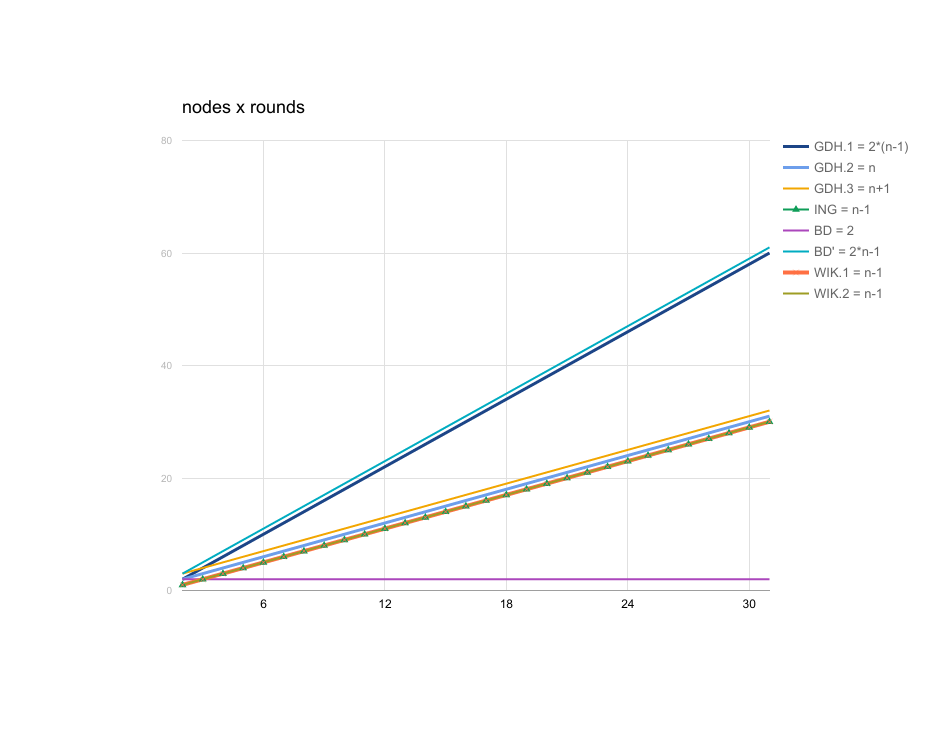Number of rounds, ends up describing the amount of time needed for n nodes to exchange the messages. As a smaller number of rounds means that more messages can be exchanged concurrently, in the same round.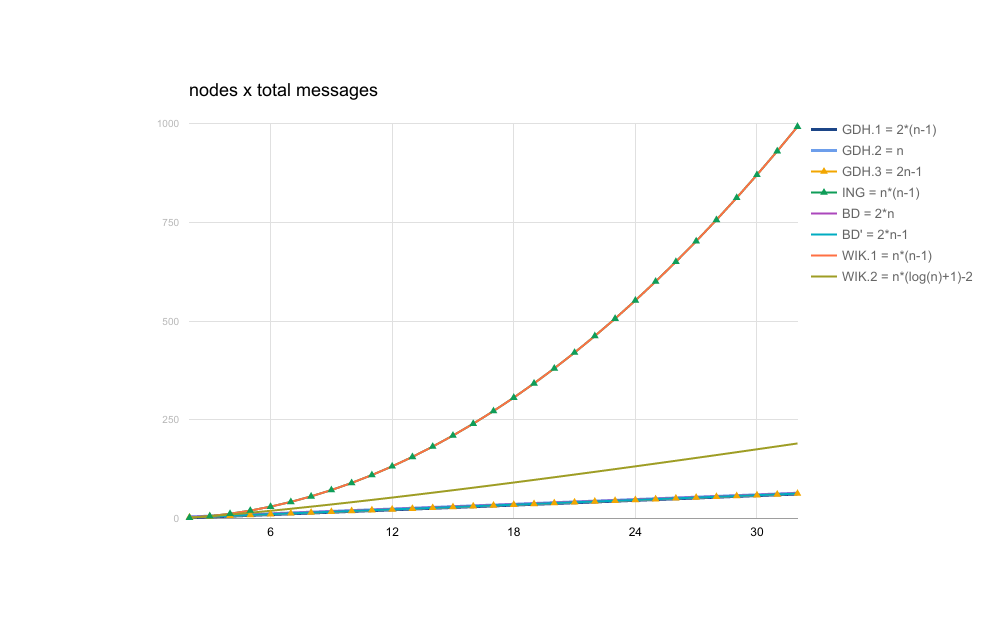Total messages is simply the total number of transmitted messages in the network.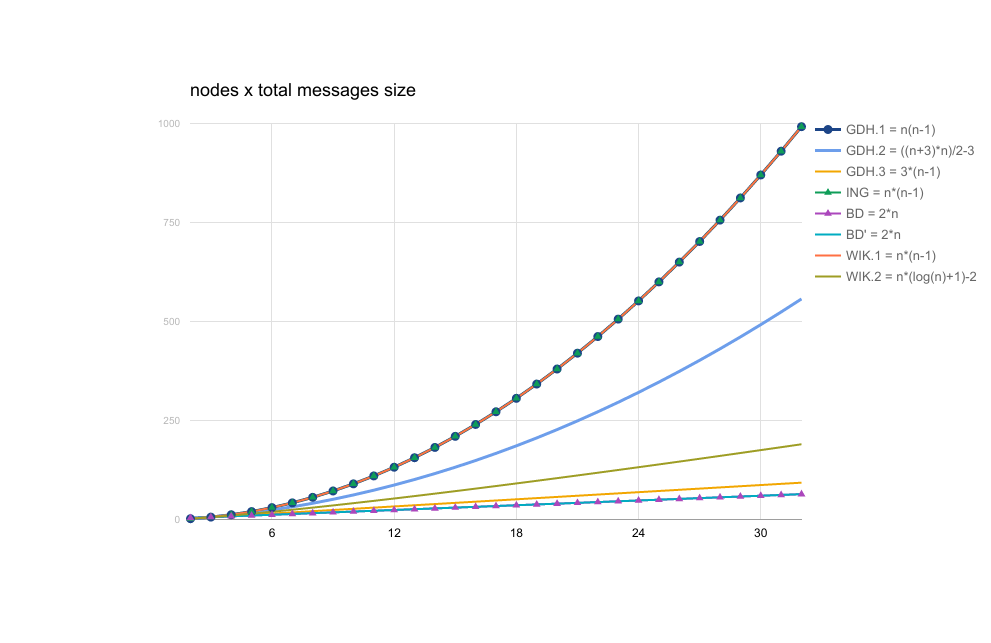Total message size, is the sum of the size of all transmitted messages. Some messages can be simple and small, but some messages can be larger, carrying more information.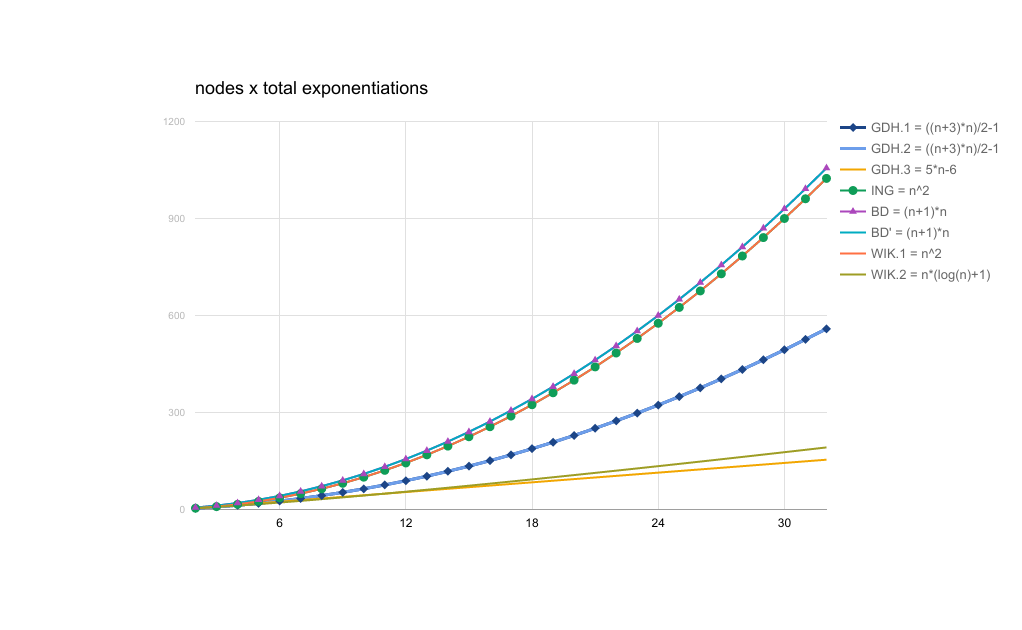Total exponentiations, is the sum of the amount of exponentiations performed by every node.

### Conclusions for Task 2

Based upon the above metrics, we've opted for GDH.3 as our algorithm of choice, because it performed well in every metric, and it has a peculiar feature on the number of exponentiations. For this algorithm every node has only to perform (at max) 4 exponentiations, except one. As we can choose which node needs to perform more work, we can simply choose the gateway, that doesn't need to depend upon a battery as power source. The inner workings of GDH.3 can be found in Steiner[].

WIK.2 has also performed rather well on the metrics, even though it is a simpler algorithm, it deserves some recognition.

## Task 3 - Simulate the chosen algorithm

We made a simple Python program to simulate each stage of the GDH.3 algorithm, the code can be found here. The major behaviors of the algorithm can be observed, and also some implementation details that will need to be considered as we progress.

1. We will need some more messages in the initial phase, than shown in Steiner[]. Because in the first moment the nodes don't even know that a group-key-exchange was initiated, and they need to take part in it, also they don't know which are the other nodes taking part, therefore don't know which are the "next" node that they should forward their messages to. The node described as Mn-1 in the paper also needs to know about every other node.
2. Similarly to the previous detail, what's the use of the group if the interested nodes don't know every other node in the group? The gateway should also pass this information on the initial phase. OR have a service to answer, based of "group id", what are the nodes in the group.
3. The nodes need to pass information about which phase the group-key-exchange is to know how to answer to subsequent messages. OR store locally which is the last stage of the exchange that they answered for, being able to know which should be the next; the "group id" could also enter here allowing to a node to participate in multiple key-exchahnges at the same time
4. We have also took into account some simple security problems that could arise. No big measures need to be taken, as it seems that the only problems that could arise, would arise in any chosen algorithm, being it for a group or the already implemented Diffie-Hellman. Attacks could only consist of replay, or man-in-the-middle that would be invalidated by the cryptography based on time seen in Poly.

### Conclusions for Task 3

Unfortunately the timing of the first test was a burden for us, and we couldn't implement the simulation on C++ using the interface of methods seen in EPOS. Anyway we could observe some behaviors that were not clear by just reading the paper, and will be very helpful to already have thought out possible solutions for them.

The "group id", underlined on the text above, seem like a good solution to lessen the size of the messages, so we don't need to pass all the nodes of the group to every other node, nor keep the state of key-exchange in the messages.

It was also observed that it would be hard, and out of this work's scope, to calculate the optimal order of the key-exchange based on each node geographic position and range. Since traffic in the network is routed through a reachable node when the destination is not in range of the source of a message, ordering the nodes based on their location and range could minimize the routing needed, thus reducing network traffic and battery consumpion.

## Task 4 - Algorithm Implementation

Node state:

GDH_WAITING_EXP
GDH_WAITING_POP
GDH_WAITING_FINAL
GDH_WAITING_GW

Message types:

GDH_SETUP_FIRST
GDH_SETUP_INTERMEDIATE
GDH_SETUP_LAST

GDH_ROUND
GDH_RESPONSE

### Setup Messages

The setup messages are the initial messages in the algorithm. They are all sent by the gateway to tell each node which type they are and which state they should be.

• GDH_SETUP_FIRST:

Content: Group id, base, q, next.
This is the type of the message sent to the first node, the one that will start by calculating the modular exponentiation with its own private key. It will send the result, their partial key, to the next node specified in the message and change its state to GDH_WAITING_POP.

• GDH_SETUP_INTERMEDIATE:

Content: Group id, base, q, next.
This is the type of message sent to all the intermediate nodes. This will change their state to GDH_WAITING_EXP. The intermediate nodes need to wait until the first node or another intermediate node sends them their partial key. This will be explained in the GDH_ROUND message type.

• GDH_SETUP_LAST:

Content: Group id, base, q, list<node> next.
This is the type of message sent to the last node. This will change its state to GDH_WAITING_EXP. The last node needs to wait until another node (first or intermediate) sends it their partial key. The list of nodes next contains all the nodes, including the gateway, except itself.

### Key Distribution Messages

The key distribution messages are sent after the setup messages and are used to calculate the key.

• GDH_ROUND:

Content: Group id, partial key.
Sent by: First and intermediate nodes.
The GDH_ROUND message is the basic partial key exchange message. The behaviour of the nodes when they receive a GDH_ROUND message is explained in the state machine diagram of each node, but basically, a node receives a partial key, calculates the modular exponentiation of that partial key to the power of its own private key (randomly generated) and sends the result to the next node.

Content: Group id, partial key.
Sent by: Last node and Gateway.
The GDH_BROADCAST message is sent by the last node and the gateway when they need to send partial keys to all nodes. This is used by the last node to send the nodes the partial keys so they can remove their private keys and by the gateway to send the nodes the last round of partial keys.

• GDH_RESPONSE:

Content: Group id, partial key.
Sent by: First, intermediate and last nodes.
The GDH_RESPONSE message is sent from all the nodes to the gateway containing a partial key that is exponentiated by everyone except the source of the message itself and the gateway. This is calculated from the partial key received in the GDH_BROADCAST from the last node exponentiated to the power of the multiplicative inverse of the current node’s private key.

### Node States

• GDH_WAITING_EXP

Possible to: Intermediate and last nodes.
The GDH_WAITING_EXP state represents the state of the node when it is waiting a partial key so it can exponentiate that to the power of its private key.

• GDH_WAITING_POP

Possible to: First and intermediate nodes.
This is the state the first and intermediate nodes stay after they have already done their initial exponentiation but are still waiting for the round to finish and the last node to send them the partial key again. Pop refers to the fact that the act of exponentiating the partial key received from the last node by the multiplicative inverse of the current node’s private key is the same as removing the private key from the partial key, so popping it out.

• GDH_WAITING_GW

Possible to: Gateway
This is the state the gateway stays when it is waiting for all the nodes’ exponentiation.

• GDH_WAITING_FINAL

Possible to: First, intermediate, last nodes.
This is the state the nodes (except the gateway) stay when they are waiting for the gateway’s final exponentiation. This is the last state before the node can compute the last exponentiation and acquire the final shared key.

### State Machines

The state machines for the nodes are described here. The notation used is described as follows:
NODE -> MESSAGE(PARAMETERS)
The node represented by the state machine(current node) received the MESSAGE message from NODE with PARAMETERS.
MESSAGE(PARAMETERS) -> NODE
The current node will send the MESSAGE message to NODE with PARAMETERS.
The new partial key is calculated using the partial key received in the previous GDH_ROUND message to the power of the private key of the current node for the GDH_ROUND message.
The new partial key is calculated using the partial key received in the previous GDH_BROADCAST message to the power of the multiplicative inverse of the private key for the GDH_RESPONSE message.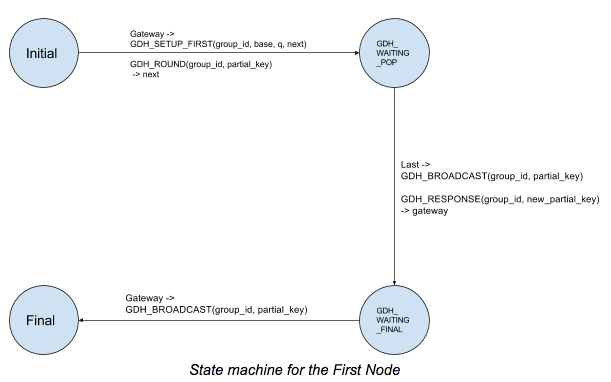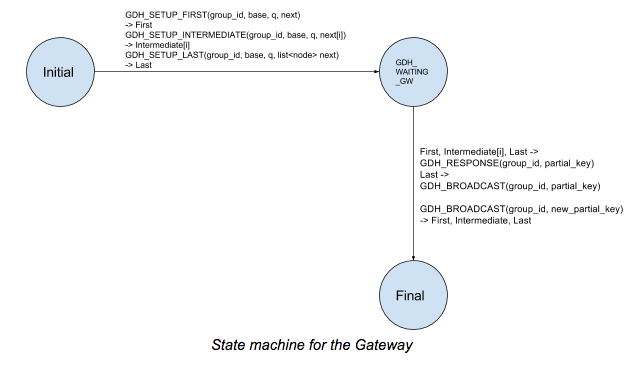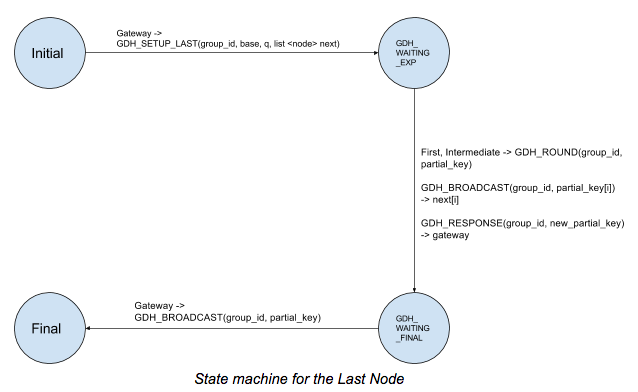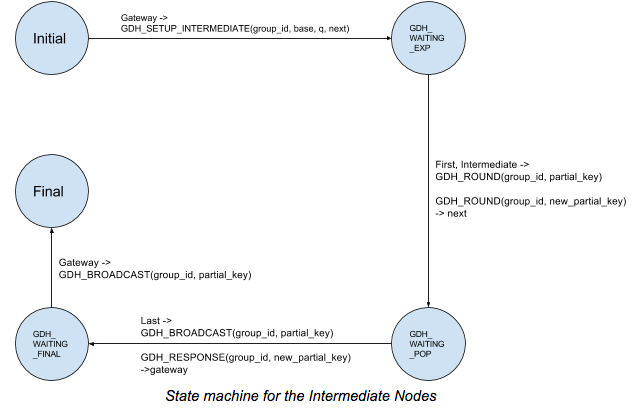### Known attacks

If an attacker sends a GDH_ROUND message with a valid group id and a random partial key, this could completely disable the actual round that is using that group id.

### Approaches to calculate the keys

Three techniques were tried, unfortunately we couldn't make Bignum or Elliptic Curves work. Here is a brief report on what went wrong:

Our first try was using a Bignum as our round key. We have two main calculations that need to be done, one is a modular exponentiation where we make (round_key**private_key) % q, and the other is to calculate the inverse of the private_key on a modulo inverse*private_key = 1 % q+1. We thought that would be interesting to make the base and q dynamic so they could be different for different groups, that leaded us into making new methods for the Bignum class.
One method mod_exp would also need a helper method, that needed to be implemented, "simple_mod" because the mod that was already available using the "barret_reduction" was hardcoded. "simple_mod" would be mostly a copy of "barret_reduction" but changing the _mod variable that would be received through a parameter. The problem was that to use "barret_reduction" we need to calculate a value u(mi) for each different mod, this calculation is not very heavy but it uses more, and more functions that would need to be coded.
The simplest description of how to code the "barret_u" is described below, as an excerpt from a comment in our code.

 p is a prime, or simply the mod l is the word size, or near the word size, usually simply 32 we can make l as large as the amount of significant bits in our BigNum b is 2 ** l k is the amount of digits needed to represent p in base b k could be calculated using floorp ,b + 1 or we could simply use b larger than p, then k is always 1 to make b larger, we just need to make l as large a the the significant bits it p the result is floor (2 * k" class="wiki wikinew text-danger tips">b (2 * k / p)

We decided to move away from it, and try using the "Elliptic_Curve_Point" from the original "Diffie_Hellman" class. We moved it to a separate file. And changed all our calls to use the "Elliptic_Curve_Point" instead of Bignum. We've also seen how using the already hardcoded base and mod would make it so much simpler to code. The good news were, the "mod_exp" equivalent was already coded, no problems there. We only needed to make the inverse work on it. After much search on Elliptic Curves and Finite Fields we could find only one example of a similar property, that could be used the same way the inverse would be used on the exponentiation. But,
it is a vague answer from 2013 on StackOverflow. It was needed more study to try to understand some of the math dialect on the answer, and our conclusion is that we only need to find "d"(that means the counting points of a elliptic curve) once, and it could be hardcoded afterwards. Here are some ways to calculate d, but most of them went over our heads, but the simple "Baby-step giant-step" seemed good enough. We precalculated the minimum and maximum possible values of "d", and would simply iterate between them to find our correct "d". This algorithm would take around 4*sqrt(q) iterations to find what we want, but our q has 128 bits, so it would take a LONG time to find it.

### Conclusions to Task 4

After taking so long over the Bignum and Elliptic Curves, we sadly decided to make it very simple using a "long long" as key instead. It certainly would not be secure in a real-world application, but it proves our points about the algorithm so we can move on to other things. I
But, we can see other possibilities that could be pursued:

1. Use the "long long" approach until the same methods are coded on Bignum, then move to Bignum. Because similar methods that he had problems on Bignum need to be implemented for "long long", but they are much simpler to code for the latter.
2. Try a better algorithm to calculate "d" for Elliptic Curves, hardcode it, and finish the rest of the calculation mentioned on the StackOverflow link. This would be the dream solution.
3. Change to a GDH algorithm that doesn't require the inverse exponentiation. That would solve a lot of trouble, and we could simply use the Elliptic Curves as they are currently.

## Task 5 - Message exchange between nodes

We started the works of this task on the previous week, as described by the automata on the previous task. The starting point of communications and message exchange was implemented on the TSTP files, with the other control messages. We also made implementations, on the app folder, that will be used as testing grounds for the messaging between the nodes. gdh_gateway and tstp_sensor can be used.
Some rough edges were smoothed out on the inner implementation of the GDH algorithm. We previously decided to use unsigned long long as our keys. But the modular exponentiation and modular inverse still needed to be detailed.
The algorithms used in the arithmetical calculations are not the best, algorithms that do not use floating point operations nor negative numbers would fulfil our needs better.

Extra work came from the fact that the original Security component was disabled and should not be used by us to implement the group diffie hellman algorithm. So we needed to create a new component, called GDH_Security and new methods do handle the messages, instead of using the already implemented ones in Security.

Another hard point was to make EPOS Motes3 communicate in the real world. More work will be put into this so we can make them communicate reliably and that a shared key is always achieved in the end.

One situation still hasn't being covered. If a GDH_ROUND message from the first node (or any other node, actually) is delivered to a note before it could be setup, it will not know how to treat it. Some kind of queue could be used to store the messages and treat them in order of arrival as soon as the setup message arrives. Extra attention will be given to this in the next weeks.

### Methods Being Used

The method that should be used by a client that wants the gateway to setup a shared key with a list of nodes is TSTP: :GDH_Security: :begin_group_diffie_hellman(Simple_List<Region::Space> nodes).
This method creates and sends all the setup messages to all nodes and, by doing that, starts the key exchange.

The message treating and most of the hard work of this protocol is done in TSTP: :GDH_Security: :update where an enum of the message type is used to differentiate the messages and correctly treat them. One extra member that we didn't predict would be needed is the node type. In the setup message, the node's type is set so it knows how to correctly treat the messages it receives, specially the GDH_ROUND messages that both the intermediate and last nodes can receive but will respond in a different way.

## Task 6 - Study how to estimate the current network load

We started our studies, using the ideas given by the professor, reading these wikipedia articles: Moving average and ARMA models. Our general opinion over the models is that they seem simple enough, and align with our work, that doesn't need to be the best prediciton/estimation, but problems could arise during the implementation phase. Similarly to what happened during the implementation of the GDH algorithm, where we needed to make some math calculations that were not simple to make using the current Bignum and Elliptic Curves implementation. We want to try and avoid falling into the same trap, so we will probably choose one of the algorithms on the Moving Average article, that are relatively simpler. Trying and avoiding the the use of hard calculations may mean that we end up not using the derivative, as suggested by the professor, but we are not sure yet. An idea to circumvent that would be using a simpler approximation(maybe using sin, cos and tan).

It is important to take a moment here to differ between two estimations we would want to do. The first, and stated on the previous paragraph, is estimating the load of all the network. The second is the estimation of how much load a key exchange would introduce to the network.

One of the problems, that we have already seen previously and became clear now, is that disposition of the nodes could influence the number of real messages sent. Because a communication between nodes A and B could become a big message passage chain(A sends to C, that sends to D, ..., that sends to B ), that would be hard to calculate. We concluded that this could be made into a future work. Anyway, we do have the best-case scenario, that would be that number we have from GDH.3, as described on a previous task. Another interesting metric would be the worst case scenario, that we imagined into the following image: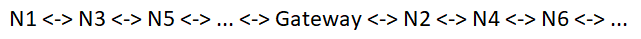Where we are trying to make a group between all the nodes of the network, and they happen to just communicate to one or two other nodes each (Represented by <->). The odd numbered nodes of the sequence to build the key are to the right of the gateway, and the even numbered to the right of the gateway. The sequence to build the key would be Gateway -> N1 -> N2 -> N3... And this disposition would make that every exchange would need to traverse half the network for a naive estimation of n*(n/2) real communications. This worst-case scenario this needs polishing, and to account for the messages on the SETUP, BROADCAST and RESPONSE phases.

One of the simpler things we could consider is simply the distance between the group and the gateway. For this estimation refer to the following image and suppose that we have a small, or at least near each other group of nodes that would want to start a key-exchange. But the group is relatively distant to the gateway.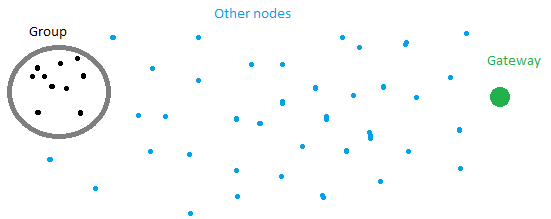It would be easy to assume that a distance d1, in hops, from one of the nodes, pertaining to the group, until the gateway, is very similar to every other distance dx of the other nodes of the group.

### Conclusions from Task 6

For estimating the load of all the network we will build our solution based on the the Moving average article, and try and avoid difficult calculations that trapped us on the first part. The algorithm will be chosen as we see fit on the next task, simulation.
Making a good estimation of the network load that will be created by the key exchange also seems hard. But we can make approximations based on best and worst case scenario, that still need some polishing, and the distance from the group to the gateway.

## Task 7 - Code simulating the network load estimations

The final decision was for the simple moving average with variable parameters. Our implementation will have 2 parameters: sample time and window size.
Sample time is the amount of time recorder as a sample. So all messages that went into the network during one second will be recorded as a number in the gateway. Our initial value will be one second.
Window size is the amount of samples that will be recorded in the system and will be used to compute the current average network load. Our initial value will be 60 samples.

Other possibility evaluated was cumulative moving average, because in this case we would not need to store so many values, just the old average. This method has a huge drawback, it stores values indefinitely, that is, very old values still influence the current average. This would not suit our needs of evaluating the current status of the network load because it slows the average movement the longer we keep the calculating it using the same old average.

We believe that the best values for sample time and window size could be discovered experimentally in a production environment, because they are context dependent. The occurence of very long cycles, where peaks are tens of minutes apart, would influence for bigger window size values.

## Task 8 - Coding the network estimation on EPOS

We've started this task by making a new Component o TSTP, the Messages_Statistic. This Component will sit alongside our already created GDH_Security Component. This new component will be responsible took keep track of the amount of messages that were received on the last samples.
As discussed on the last Task, we have a sample_time and a window_size. And we've decided to implement the sampling using an integer, therefore for every message received the sample count increases, until the the current sample ends. After that the sample is added to a FIFO Queue of maximum size window_size. The expected behavior is simple: when we add a new sample, that increases our windows queue, if the queue is larger than window_size, we remove the oldest value.
The moving average tidbit can be easily changed and experimented upon. During our experiments we've really liked the following algorithm:

 'value' is the value of the last sample that is not put into the window yet 'cur_window_average' is the average of all the values currently on the window 'alpha' is a coeficient that may be experimented upon, we use it as 0.5 'new_value' = (1-'alpha') * 'cur_window_average' + 'alpha' * 'value' 'new_value' is appended to the window, could implicitly drop an older value

It is very simple but it has nice degree of flexibilty because of the 'alpha' value. Also the 'alpha' value becomes another new parameter that can be changed, together with 'sample_time' and 'window_size'.

## Task 9 - Presentation

During the last week we have been preparing the last part of this work, the presentation. We condensed most of the information of this document onto ~35 slides that can be found here.
Also, using the application code that we have been using to test our components, we can show the new functionalities working. We've prepared a video showing it, and it can be found here.
The final version of the code can be found and downloaded from here https://github.com/nicolas0p/Group-Diffie-Hellman(@97c6319).

We also include here a list of further work that could improve some edges of our work:

1. Optimize the order of the nodes on the key-exchange, using their geographic positions.
2. Ignore copies of key-exchange messages instead of recalculating
3. Enforce the use of statistics when starting a key-exchange
4. Support the setup of multiple groups
5. Improve the load estimation algorithm